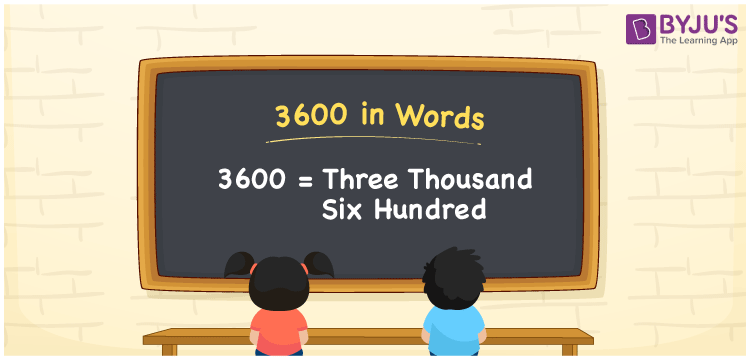# 3600 in words

3600 in words is written as Three Thousand Six Hundred. In the number 3600, 3 represents the place value of thousand and 6 has the place of hundred. The article on Place Value will get you more details. The number 3600 is used in expressions that relate to count, money, distance, length and others. Let us consider an example for the number 3600. “An advertisement was put up on a hoarding, for a flat that measured 3600 sq feet. The price per foot was Three Thousand Six Hundred rupees”

 3600 in words Three Thousand Six Hundred Three Thousand Six Hundred in Numbers 3600

## 3600 in English Words## How to Write 3600 in Words?

We can convert 3600 to words using a place value chart. The number 3600 has 4 digits, so let’s make a chart that shows the place value up to 4 digits.

 Thousands Hundreds Tens Ones 3 6 0 0

Thus, we can write the expanded form as:

3 × Thousand + 6 × Hundred + 0 × Ten + 0 × One

= 3 × 1000 + 6 × 100 + 0 × 10 + 0 × 1

= 3600

= Three Thousand Six Hundred.

3600 is the natural number that is succeeded by 3599 and preceded by 3601.

3600 in words – Three Thousand Six Hundred.

Is 3600 an odd number? – No.

Is 3600 an even number? – Yes.

Is 3600 a perfect square number? – Yes. (60 × 60 ).

Is 3600 a perfect cube number? – No.

Is 3600 a prime number? – No.

Is 3600 a composite number? – Yes.

## Solved Example

1. Write the number 3600 in expanded form

Solution: 3 × 1000 + 6 × 100 + 0 × 10 + 0 × 1

We can write 3600 = 3000 + 600 + 0 + 0

= 3 × 1000 + 6 × 100 + 0 × 10 + 0 × 1

## Frequently Asked Questions on 3600 in words

Q1

### How to write 3600 in words?

3600 in words is written as Three Thousand Six hundred.
Q2

### Is 3600 a perfect square number?

Yes. 3600 is a perfect square number. 60 multiplied by itself gives 3600.
Q3

### Is 3600 divisible by 10?

Yes. 3600 is divisible by 10.